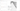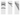# Modeling

1-D Seismic Modeling
Simulate surface waves, refracted and reflected waves for a layered velocity model with analytic solutionsWave types
• Reflected and refracted P-waves
• Reflected and refracted S-waves
• Fundamental and higher-mode surface waves
• Air wave
Modeling
• Input layered velocity model
• Select wave types
• Set up geometry
• Specify source location and wavelet
• Set sampling parameters
• Simulate all types of waves with analytic solutions
Plotting
• Velocity model
• Time-distance (T-X) curves
• Dispersion curves of fundamental and higher modes
• Seismograms